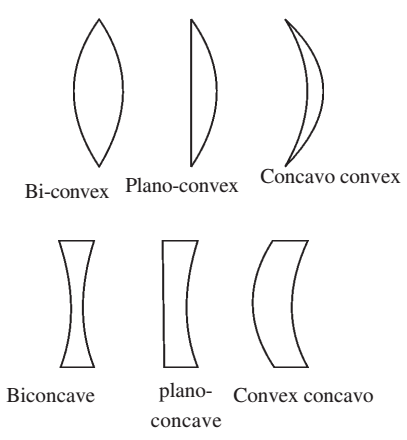# Reflection Through Lenses

A lens is a thin piece of transparent material (usually glass) having two surfaces, one or both of which are curved (mostly spherical). Lenses are mainly of two types: convex lens and concave lens.

### Types of Lenses### Basic Terms

Thin lens: If the thickness of a lens is negligible in comparison to the radii of curvature of its curved surfaces, the lens is referred to as a thin lens.

Principal axis is the line joining the centres of curvature of two surfaces of the lens.

Optical centre is the point at the centre of the lens situated on the principal axis. The rays passing through the optical centre do not deviate.

Principal focus is the point at which rays parallel and close to the principal axis converge to or appear to diverge. It is denoted by F. Rays of light can pass through a lens in either direction. So every lens has two principal focii, one on its either side.

Focal length is the distance between the optical centre and the principal focus. OF is focal length (f). As per the sign convention, OF is positive for a convex lens and negative for a concave lens.

Focal plane is the plane passing through the focus of a lens perpendicular to its principal axis.